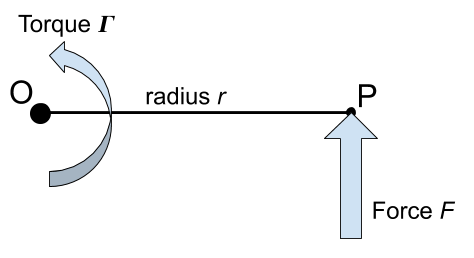Question #192

# What is the formula to calculate the torque generated by a force applied perpendicular to the radius?

Merged questions
AnswerThe formula to calculate the torque generated by a force applied perpendicular to the radius is:

﻿﻿

With :

• ﻿﻿ is the torque expressed in newtons-meters [N.m]
• ﻿﻿ is the force expressed in newtons [N]
• ﻿﻿ is the radius expressed in meters [m], note that ﻿﻿ , the length of the OP segment.

6 events in history
Answer by Alphonsio 09/18/2020 at 05:48:37 PMThe formula to calculate the torque generated by a force applied perpendicular to the radius is:

﻿﻿

With :

• ﻿﻿ is the torque expressed in newtons-meters [N.m]
• ﻿﻿ is the force expressed in newtons [N]
• ﻿﻿ is the radius expressed in meters [m], note that ﻿﻿ , the length of the OP segment.

Answer by Alphonsio 09/18/2020 at 05:46:38 PMThe formula to calculate the torque generated by a force applied perpendicular to the radius is:

﻿﻿

With :

• ﻿﻿ is the torque expressed in newtons-meters [N.m]
• ﻿﻿ is the force expressed in newtons [N]
• ﻿﻿ is the radius expressed in meters [m], note that ﻿﻿ , the length of the OP segment.

Answer by Alphonsio 09/18/2020 at 05:42:43 PMThe formula to calculate the torque generated by a force applied perpendicular to the radius is:

﻿﻿

With :

• ﻿﻿ is the torque expressed newtons-meters [N.m]
• ﻿﻿ is the force expressed in newtons [N]
• ﻿﻿ is the radius expressed in meters [m], note that ﻿﻿ , the length of the OP segment.

Answer by Alphonsio 09/18/2020 at 05:36:04 PMThe formula to calculate the torque generated by a force applied perpendicular to the radius is:

﻿﻿

With :

• ﻿﻿ is the torque expressed newtons-meters [N.m]
• ﻿﻿ is the force expressed in newtons [N]
• ﻿﻿ is the radius expressed in meters [m], note that ﻿﻿ , the length of the OP segment.
Answer by Alphonsio 09/18/2020 at 05:35:02 PMThe formula to calculate the torque generated by a force applied perpendicular to the radius is:

﻿﻿

With :

• ﻿﻿ is the torque expressed newtons-meters [N.m]
• ﻿﻿ is the force expressed in newtons [N]
• ﻿﻿ is the radius expressed in meters [m], note that ﻿﻿ , the length of the OP segment.
Question by Alphonsio 09/18/2020 at 05:33:00 PM
What is the formula to calculate the torque generated by a force applied perpendicular to the radius?

Icons proudly provided by Friconix.